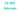# CS502- Design and Analysis of Algorithms Quiz

0
148CS502- Design and Analysis of Algorithms Quiz

1. For the sieve technique we solve the problem,

(Handsouts Page 35 Line 4)

2. We do sorting to,

(Handsouts Page 39 Line 6)

3. The reason for introducing Sieve Technique algorithm is that it illustrates a very important special case of,

(handsouts page 34 )

4. In Sieve Technique we don’t know which item is of interest

handsouts page 34

CS502- Design and Analysis of Algorithms Quiz

5. In the analysis of Selection algorithm, we make a number of passes, in fact it could be as many as,

(handsouts page 37) )

6. Divide-and-conquer as breaking the problem into a small number of pivot Sieve